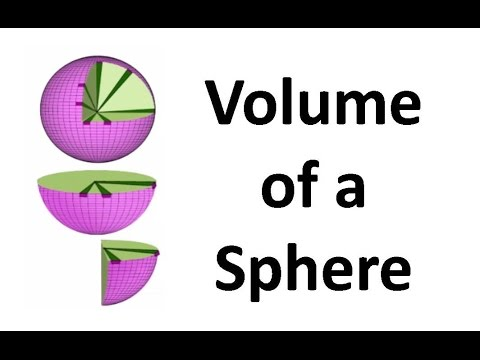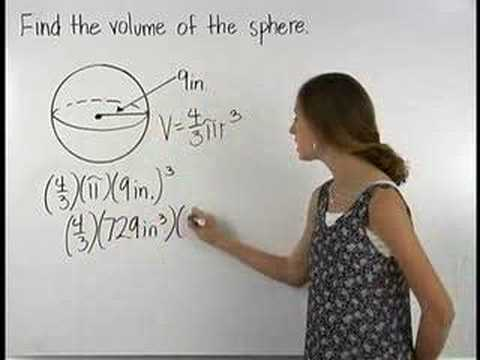## Sphere and Hemisphere

Subject: Compulsory Mathematics

#### Overview

The sphere is a solid object whose each point in the outer surface is equidistance from the fixed point inside it. Such a fixed point is called the centre of the sphere. The constant distance is called the radius of the sphere. Solid object such as globe, volleyball, toy ball, table tennis ball, marble etc are the example of sphere.
##### Sphere and Hemisphere

We are so much familiar with spherical objects. The sphere is also a solid object whose each point in the outer surface is equidistance from the fixed point inside it. Such a fixed point is called the centre of the sphere. The constant distance is called the radius of the sphere. A solid object such as a globe, volleyball, toy ball, table tennis ball, marble etc is the example of a sphere.The figure as shown alongside is a sphere. The fixed point 'O' inside it is the centre which is equidistance from each point P on the surface of the sphere. So, OP = r is the radius of the sphere.If we cut a sphere through its diameter, there are two half spheres called the hemisphere and the cross section is called the great circle. The radius of the sphere is same as the radius of the great circle. The centre of the sphere and its great circle. The centre of the sphere and its great circle is same.### Surface area of sphere

The surface area of a sphere is the area of its outer part, which is a smooth curved surface.

The surface area of a sphere is given by SA = 4πr2 where r is the radius of the sphere. The total surface area of hemisphere = 2πr2+ πr2 = 3πr2 square unit.

 Note Curved surface area of hemisphere = 2$\pi$r2square units. The surface area of a sphere (SA) = $\pi$d2, if the diameter is given.

### Volume of sphereThe volume of a sphere means the space that it occupies. We can measure the volume of sphere experimentally. Fill up the measuring cylinder with the water level in the cylinder. The difference of two levels is the volume of the sphere.

#### Alternatively

Choose a sphere of given diameter (d) =10 cm (say).
Immerse of the whole sphere into the water in the measuring cylinder, the water level is raised by 523.33 ml. Therefore its volume is 523.33 cm3. From this experiment,
The diameter (d) = 10cm
The volume of sphere (V) = 523.33 cm3
Let us make the ratio

\begin{align*} 6V:d^3 &= \frac {6V} {d^3} \\ &= \frac {6 \times 523.33} {10^3} \\ &= \frac {3140} {1000} \\ &= 3.14 \end{align*}

$$(\because \pi = \frac {22} {7} = 3.14)$$

\begin{align*} \therefore \frac {6V} {d^3} &= \pi \\ or, V &= \frac {\pi (2r)^3} {6} \\ &= \frac {4 \pi r^3} {3} \\ \end{align*}

$\therefore \text {Volume of a sphere} (V) = \frac {4 \pi r^3} {3} = \frac {\pi d^3} {6} \text {cubic units.}$

##### Things to remember
1. Curved surface area of hemisphere = 2$\pi$r2square units.
2. The surface area of a sphere (SA) =d2, if the diameter is given.
3. Volume of a sphere(v) = $\frac{4\ (\pi) r^3}{3}$ = $\frac{\ (\pi)d^3}{6}$ cubic units.
• It includes every relationship which established among the people.
• There can be more than one community in a society. Community smaller than society.
• It is a network of social relationships which cannot see or touched.
• common interests and common objectives are not necessary for society.
##### Videos for Sphere and Hemisphere##### Formulas to calculate volume of a sphere and hemisphere##### Volume of a Sphere - MathHelp.com - Math Help

Here,

r = 15 cm

\begin{align*} \text{Total Surface Area of hemisphere} &= 3{\pi}r^2\\ &= 3 \times \frac {22}7 \times 15 \times 15\\ &= \frac {14850}{7}\\ &= 2121.43 cm^2_{Ans}\\ \end{align*}

Surface Area of the sphere (A) = 616 cm2

radius of the sphere (r) = ?

By formula,

A = 4$\pi$r2

or, r2 = $\frac A{4\pi}$

or, r2 = $\frac {616 \times 7}{4 \times 22}$

or, r2 = 49

∴ r = 7cmAns

Suppose,

Then,

Surface Area of Sphere = 4$\pi$r2

or, $\pi$ = 4$\pi$r2

or, r2 = $\frac {\pi}{4\pi}$

or, r2 = $\frac 14$

or, r = $\sqrt {\frac 14}$

∴ r = $\frac 12$cmAns

Here,

r = $\frac {42 cm}{2}$ = 21 cm

\begin{align*} \text{Volume of the hemisphere (V)} &=\frac 23{\pi}r^3\\ &= \frac 23 \times \frac {22}7 \times (21)^3\\ &= 44 \times (21)^2\\ &= 19404 cm^3_{Ans}\\ \end{align*}

Here,

Volume of marble (V) = $\frac {\pi}6cm^3$

or, $\frac 43$ $\pi$r3 = $\frac {\pi}6cm^3$

or, r3 = $\frac {\pi}6$ $\times$ $\frac 3{4\pi}cm^3$

or, r3 = $\frac 1{2^3}cm^3$

∴ r = $\frac 12cm$

Hence, the diameter of the marble = 2 $\times$ r = 2 $\times$ $\frac 12$ cm = 1 cmAns

Let r be the radius of the sphere.

Volume = $\frac 43$$\pi$r3

or, 38808 = $\frac 43$ $\times$ $\frac {22}7$ $\times$ r3

or, r3 = 38808 $\times$ $\frac {21}{88}$

or, r3 = 441 $\times$ 21

or, r3 = (21)3

∴ r = 21Ans

Here,

Volume of sphere = $\frac {3773}{21}cm^3$

or, $\frac 43$$\pi$r3 = $\frac {3773}{21}cm^3$

or, $\frac 43$ $\times$ $\frac {22}7$ $\times$ r3 = $\frac {3773}{21}cm^3$

or, $\frac {88}{21}r^3$ = $\frac {3773}{21}cm^3$

or, r3 = $\frac {3773}{21}$ $\times$ $\frac {21}{88}cm^3$

or, r3 = $\frac {343}{8}cm^3$

or, r3 = ($\frac 73$cm)3

∴ r = $\frac 72$cm

Hence, Circumference of sphere = 2$\pi$r = 2 $\times$ $\frac {22}7$ $\times$ $\frac 72$ cm = 22 cmAns

Here,

Volume of spherical solid = $\frac 43$${\pi}cm^3$

or, $\frac 43$${\pi}r^3$ = $\frac 43$${\pi}cm^3$

or, r3 = $\frac {\frac {4\pi}{3}}{\frac {4\pi}{3}}cm^3$

or, r3 = 1 cm3

∴ r = 1 cm

Hence, the radius of spherical solid is 1 cm.Ans

Here,

Volume of sphere (V) = 36${\pi}cm^3$

Diameter of a sphere (d) = ?

We know that,

V = $\frac 16$${\pi}d^3$

or, d = $\sqrt \frac{6V}{d}$

or, d = $\sqrt \frac {6 \times 36\pi}{\pi}$

or, d = $\sqrt {6 \times 6 \times 6}$

∴ d = 6cm

\begin{align*} \text{The Surface Area (A)} &= {\pi}d^2\\ &= \frac {22}7 \times (6cm)^2\\ &= \frac {22}7 \times 36 cm^2\\ &= 113.14cm^2_{Ans}\\ \end{align*}

Here,

Volume (V) = $\frac {1372\pi}{3}cm^3$

Total Surface Area (S) = ?

By Formula,

V = $\frac 43$${\pi}r^3$

or, $\frac {1372\pi}{3}$ =$\frac 43$${\pi}r^3$

or, r3 =$\frac {1372\pi}{3}$ $\times$ $\frac 3{4\pi}$

or, r3 = 343

or, r3 = 73

∴ r = 7

We know that,

\begin{align*} S &= 4{\pi}r^2\\ &= 4 \times \frac {22}7 \times 7^2 cm^2\\ &= 616 cm^2_{Ans}\\ \end{align*}

Here,

Surface Area of sphere = 616 cm2

or, 4${\pi}r^2$ = 616 cm2

or, ${\pi}r^2$ = $\frac {616}4 cm^2$

∴${\pi}r^2$ = 154 cm2

We know that,

\begin{align*} \text{Total Surface Area of a hemisphere} &= 3{\pi}r^2\\ &= 3 \times 154 cm^2\\ &= 462 cm^2_{Ans}\\ \end{align*}

Here,

Total Surface Area of hemisphere (S) = 243 ${\pi}cm^2$

Volume (V) = ?

We know that,

S = 3${\pi}r^2$

or, 243$\pi$ = 3${\pi}r^2$

or, r2 = $\frac {243\pi}{3\pi}$

or, r2 = 81

∴ r = 9 cm

Now,

\begin{align*} V &= \frac 23 {\pi}r^3\\ &= \frac 23 \times \frac {22}7 \times 9^3\\ &= 1527.43 cm^3_{Ans}\\ \end{align*}

Here,

4${\pi}r^2$ = $\frac 1{4\pi}$

or, r2 = $\frac 1{(4\pi)^2}$

∴r = $\frac 1{4\pi}$

Now,

\begin{align*} \text{Volume (V)} &= \frac 43 {\pi}r^3\\ &= \frac {4\pi}3 (\frac 1{4\pi})^3\\ &= \frac 13 \times \frac 1{16\pi^2}\\ &= \frac 1{44\pi^2}cm^3_{Ans}\\ \end{align*}

Here,

r1 = $\frac {6cm}2$ = 3cm

r2 = $\frac {8cm}2$ = 4cm

r3 = $\frac {10cm}{2}$ = 5cm

Suppose,

radius of new sphere = R

Now,

Volume of new sphere = sum of the volume of first, second and third spheres

or, $\frac 43$${\pi}R^3$ = $\frac 43$${\pi}r_1^3$ +$\frac 43$${\pi}r_2^3$ +$\frac 43$${\pi}r_3^3$

or, R3 = r13 + r23 + r33

or, R3 = (33 + 43 + 53)

or, R3 = 27 + 64 + 125

or, R3 = 216

or, R = $\sqrt {216}$

∴ R = 6 cm

Hence, the diameter of new sphere (d) = 2R = 2 $\times$ 6 cm = 12 cmAns

\begin{align*} \text{Volume of small ball} &= \frac 43 {\pi}r^3\\ &= \frac 43 {\pi}1^3\\ &= \frac 43 {\pi}cm^3\\ \end{align*}

\begin{align*} \text{Radius of big ball} &= \frac {8cm}2\\ &= 4 cm\\ \end{align*}

\begin{align*} \text{Volume of big ball} &= \frac 43 {\pi}r^3\\ &= \frac 43 {\pi}4^3\\ &= \frac 43 {\pi}64cm^3\\ \end{align*}

Now,

\begin{align*} \text{Number of small balls that can be made from the big ball} &= \frac {\frac 43 {\pi} \times 64}{\frac 43 {\pi}}\\ &= 64_{Ans}\\ \end{align*}

Let x be the radius of the first sphere.

Then,

Radius of second sphere is $\frac r4$

Volume of first sphere (V1) = $\frac 43$ ${\pi}r^3$

Volume of second sphere (V2) = $\frac 43$ $\pi$ ($\frac r4$)3 = $\frac {4\pi}{3}$$\times$ $\frac {r^3}{64}$

Now,

$\frac {V_2}{V_1}$ = $\frac {\frac {4\pi}{3} \times \frac {r^3}{64}}{\frac 43 {\pi}r^3}$ = $\frac 1{64}$

Hence, V1 :V2 = 1 : 64Ans